# Bayesian Neural Networks Bayesian statistics An example of

• Slides: 32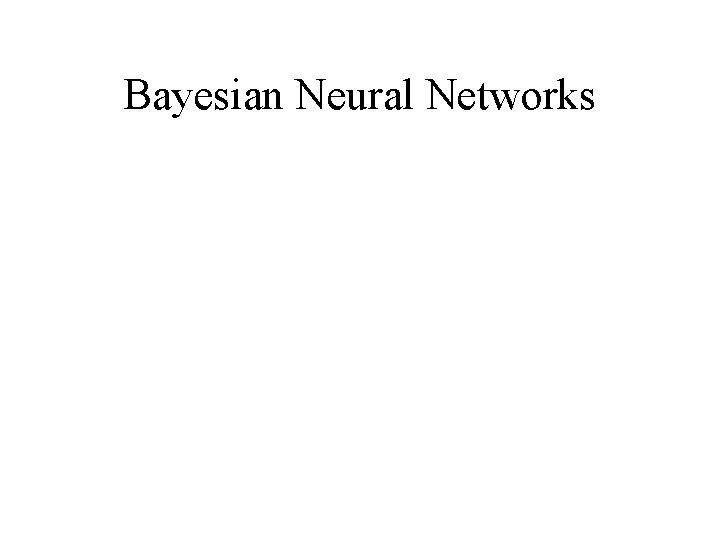Bayesian Neural NetworksBayesian statistics • An example of Bayesian statistics: “The probability of it raining tomorrow is 0. 3” • Suppose we want to reason with information that contains probabilities such as: ''There is a 70% chance that the patient has a bacterial infection''. Bayes theories rest on the belief that for everything there is a prior probability that it could be true.Priors • Given a prior probability about some hypothesis (e. g. does the patient have influenza? ) there must be some evidence we can call on to adjust our views (beliefs) on the matter. • Given relevant evidence we can modify this prior probability to produce a posterior probability of the same hypothesis given new evidence. • The following terms are used:Terms • p(X) means prior probability of X • p(X|Y) means probability of X given that we have observed evidence Y • p(Y) is the probability of the evidence Y occurring on its own. • p(Y|X) is the probability of the evidence Y occurring given the hypothesis X is true (the likelihood).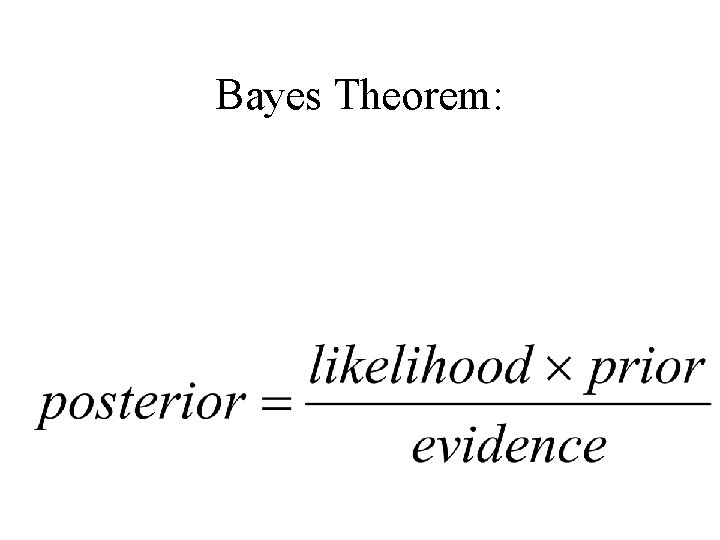Bayes Theorem:Bayes rule • We know what p(X) is - the prior probability of patients in general having influenza. • Assuming that we find that the patient has a fever, we would like to find P(X: Y) the probability of this particular patient having influenza given that we can see that they have a fever (Y). • If we don't actually know this we can ask the opposite question, i. e. if a patient has influenza, what is the probability that they have a fever?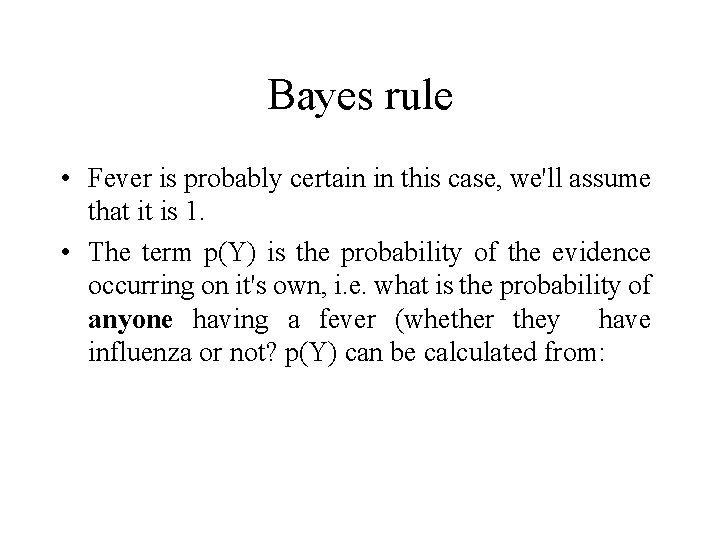Bayes rule • Fever is probably certain in this case, we'll assume that it is 1. • The term p(Y) is the probability of the evidence occurring on it's own, i. e. what is the probability of anyone having a fever (whether they have influenza or not? p(Y) can be calculated from: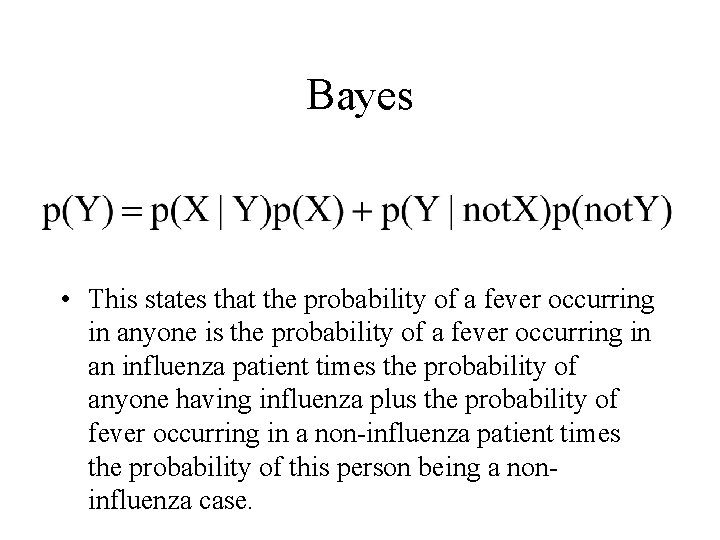Bayes • This states that the probability of a fever occurring in anyone is the probability of a fever occurring in an influenza patient times the probability of anyone having influenza plus the probability of fever occurring in a non-influenza patient times the probability of this person being a noninfluenza case.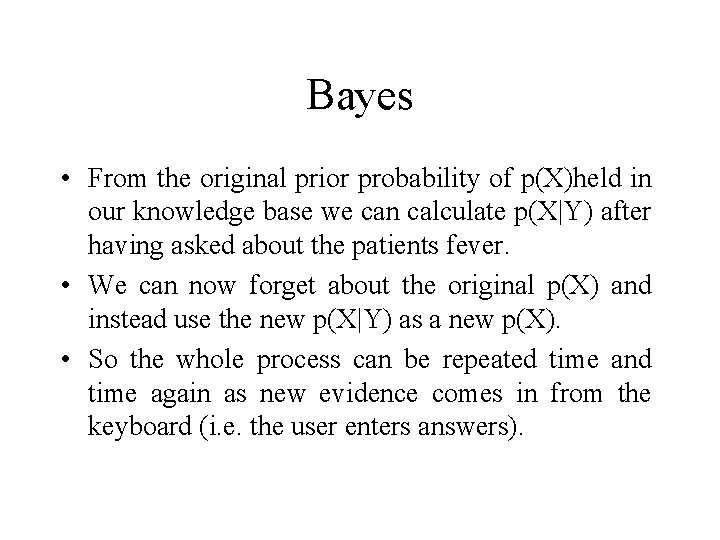Bayes • From the original prior probability of p(X)held in our knowledge base we can calculate p(X|Y) after having asked about the patients fever. • We can now forget about the original p(X) and instead use the new p(X|Y) as a new p(X). • So the whole process can be repeated time and time again as new evidence comes in from the keyboard (i. e. the user enters answers).Bayes • Each time an answer is given the probability of the illness being present is shifted up or down a bit using the Bayesian equation. • Each time a different prior probability being used which has been derived from the last posterior probability.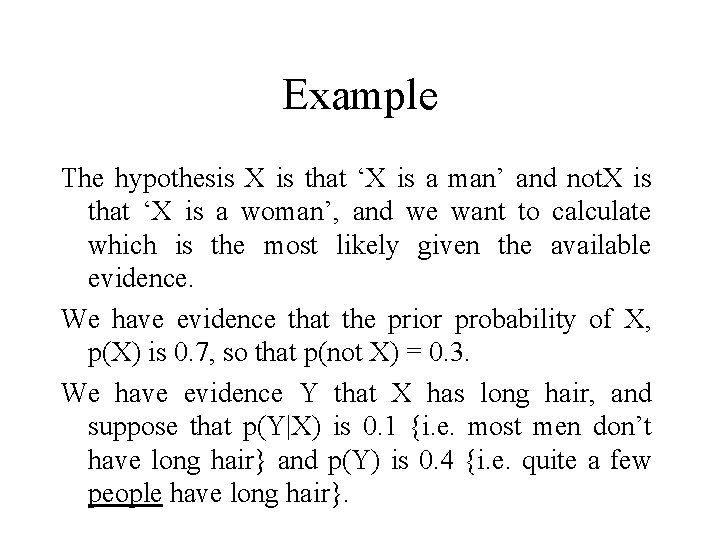Example The hypothesis X is that ‘X is a man’ and not. X is that ‘X is a woman’, and we want to calculate which is the most likely given the available evidence. We have evidence that the prior probability of X, p(X) is 0. 7, so that p(not X) = 0. 3. We have evidence Y that X has long hair, and suppose that p(Y|X) is 0. 1 {i. e. most men don’t have long hair} and p(Y) is 0. 4 {i. e. quite a few people have long hair}.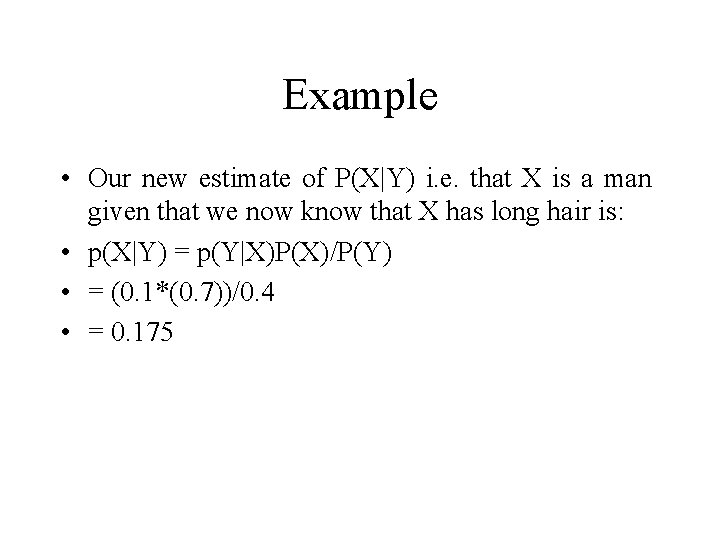Example • Our new estimate of P(X|Y) i. e. that X is a man given that we now know that X has long hair is: • p(X|Y) = p(Y|X)P(X)/P(Y) • = (0. 1*(0. 7))/0. 4 • = 0. 175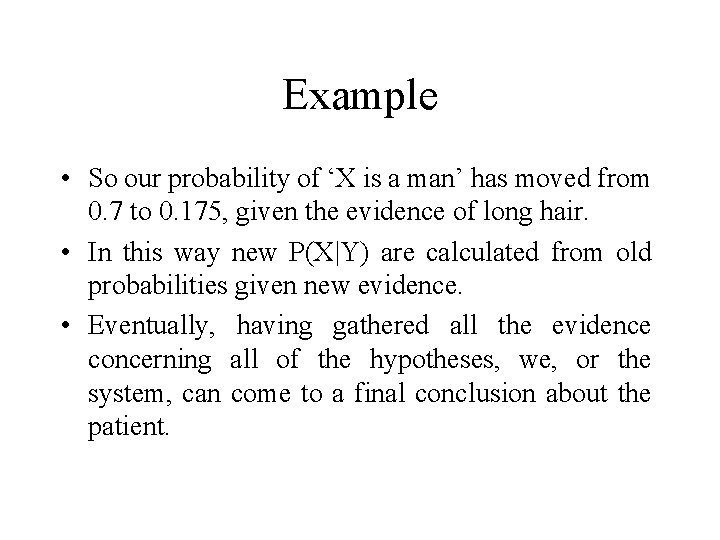Example • So our probability of ‘X is a man’ has moved from 0. 7 to 0. 175, given the evidence of long hair. • In this way new P(X|Y) are calculated from old probabilities given new evidence. • Eventually, having gathered all the evidence concerning all of the hypotheses, we, or the system, can come to a final conclusion about the patient.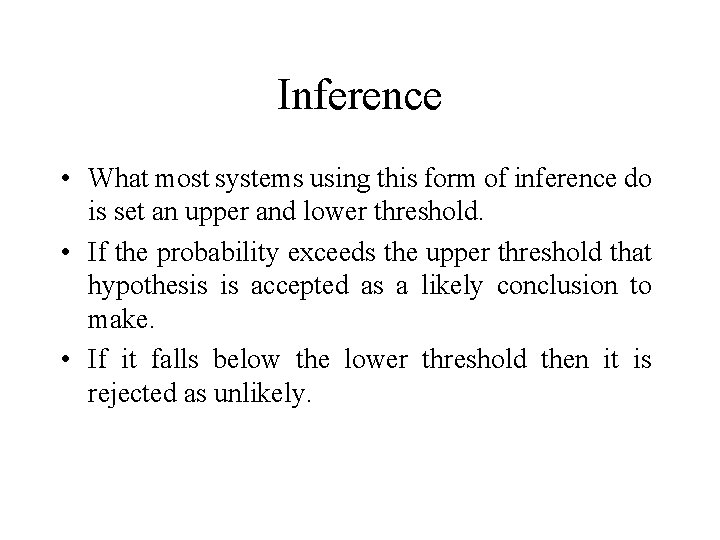Inference • What most systems using this form of inference do is set an upper and lower threshold. • If the probability exceeds the upper threshold that hypothesis is accepted as a likely conclusion to make. • If it falls below the lower threshold then it is rejected as unlikely.Problems • Computationally expensive • The Prior probabilities are not always available and are often subjective – much research in how to discover ‘informative’ prior probabilities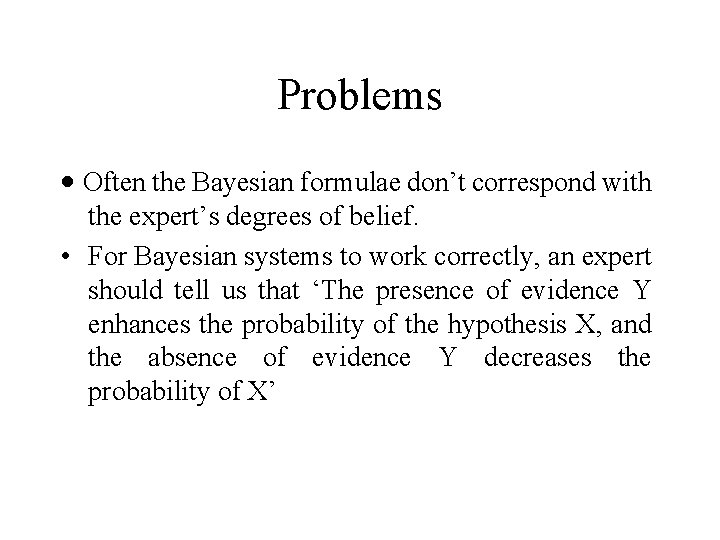Problems · Often the Bayesian formulae don’t correspond with the expert’s degrees of belief. • For Bayesian systems to work correctly, an expert should tell us that ‘The presence of evidence Y enhances the probability of the hypothesis X, and the absence of evidence Y decreases the probability of X’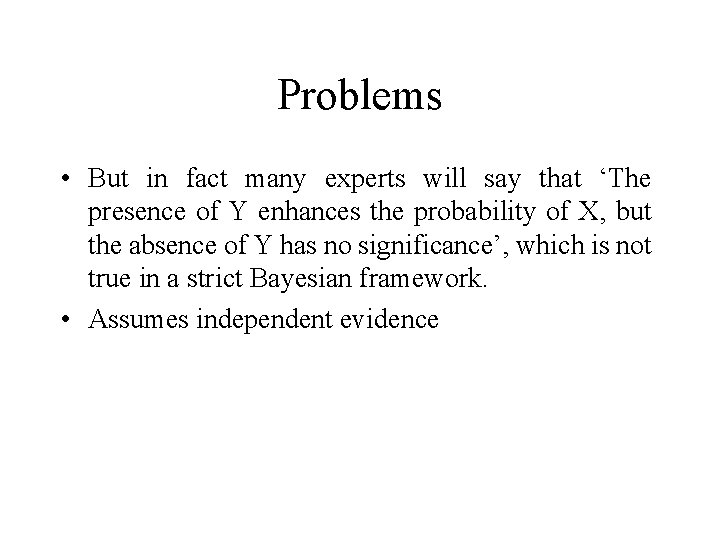Problems • But in fact many experts will say that ‘The presence of Y enhances the probability of X, but the absence of Y has no significance’, which is not true in a strict Bayesian framework. • Assumes independent evidenceBayes and NNs • Bayesian methods are often used in both statistics and Artificial Intelligence based around expert systems. • However, they can also be used with neural networks. • Conventional training methods for multilayer perceptrons (such as backpropagation) can be interpreted in statistical terms as variations on maximum likelihood estimation.Bayes and NNs • The idea is to find a single set of weights for the network that maximize the fit to the training data, perhaps modified by some sort of weight penalty to prevent overfitting. • Bayesian training automatically modifies weight decay terms so that weights that are unimportant decay to zero • In this way unimportant weights are effectively ‘pruned’ – preventing overfitting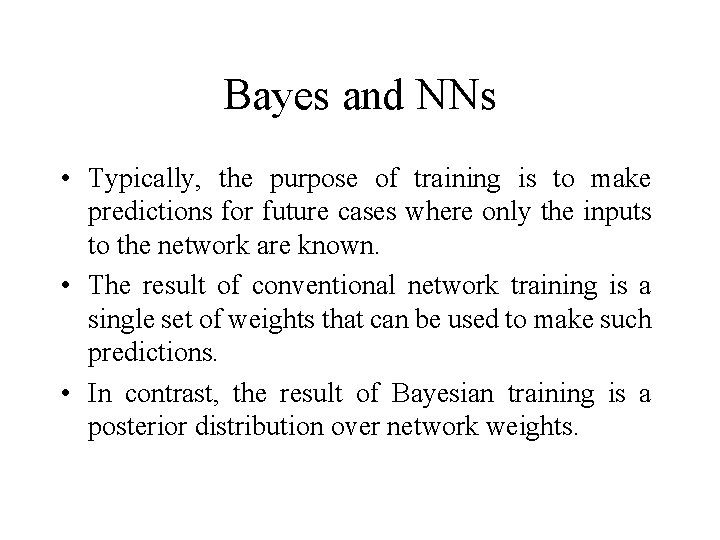Bayes and NNs • Typically, the purpose of training is to make predictions for future cases where only the inputs to the network are known. • The result of conventional network training is a single set of weights that can be used to make such predictions. • In contrast, the result of Bayesian training is a posterior distribution over network weights.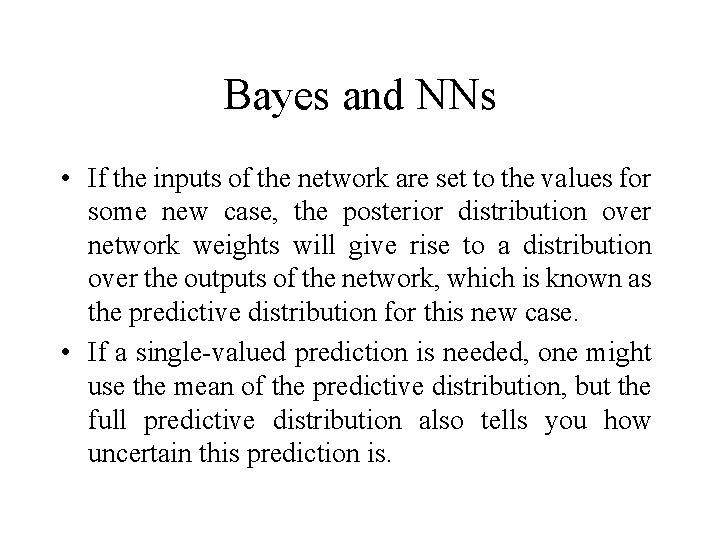Bayes and NNs • If the inputs of the network are set to the values for some new case, the posterior distribution over network weights will give rise to a distribution over the outputs of the network, which is known as the predictive distribution for this new case. • If a single-valued prediction is needed, one might use the mean of the predictive distribution, but the full predictive distribution also tells you how uncertain this prediction is.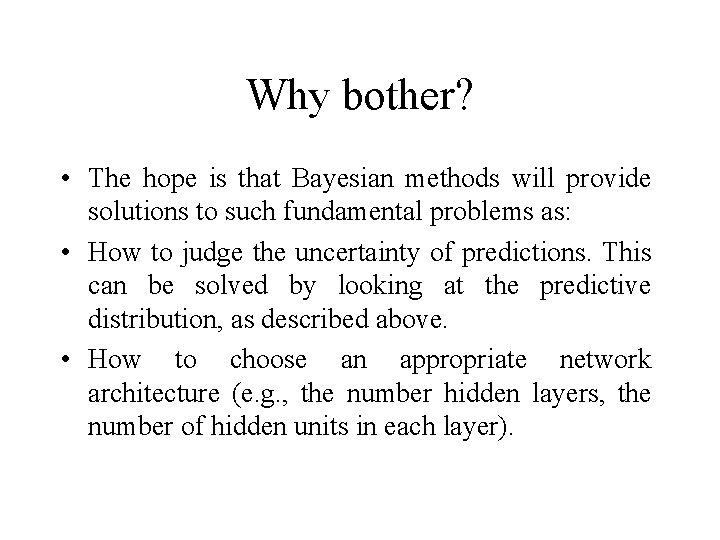Why bother? • The hope is that Bayesian methods will provide solutions to such fundamental problems as: • How to judge the uncertainty of predictions. This can be solved by looking at the predictive distribution, as described above. • How to choose an appropriate network architecture (e. g. , the number hidden layers, the number of hidden units in each layer).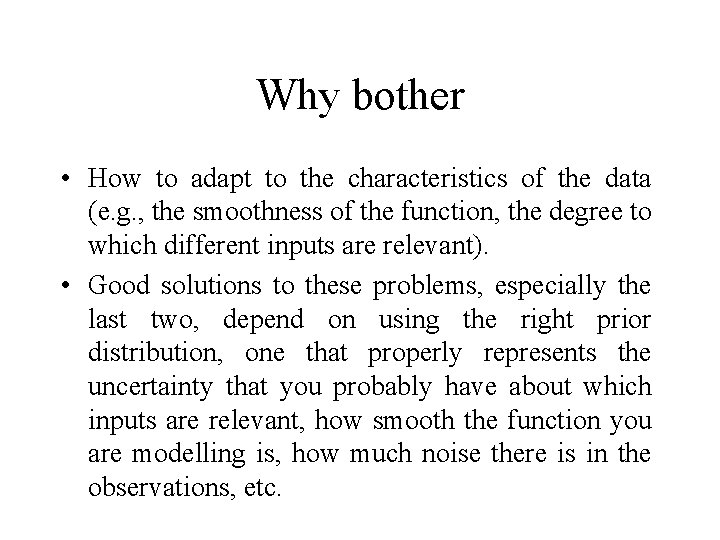Why bother • How to adapt to the characteristics of the data (e. g. , the smoothness of the function, the degree to which different inputs are relevant). • Good solutions to these problems, especially the last two, depend on using the right prior distribution, one that properly represents the uncertainty that you probably have about which inputs are relevant, how smooth the function you are modelling is, how much noise there is in the observations, etc.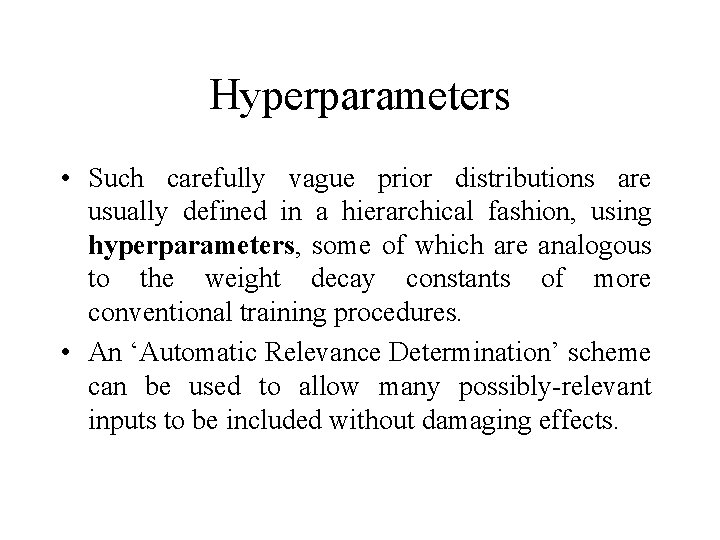Hyperparameters • Such carefully vague prior distributions are usually defined in a hierarchical fashion, using hyperparameters, some of which are analogous to the weight decay constants of more conventional training procedures. • An ‘Automatic Relevance Determination’ scheme can be used to allow many possibly-relevant inputs to be included without damaging effects.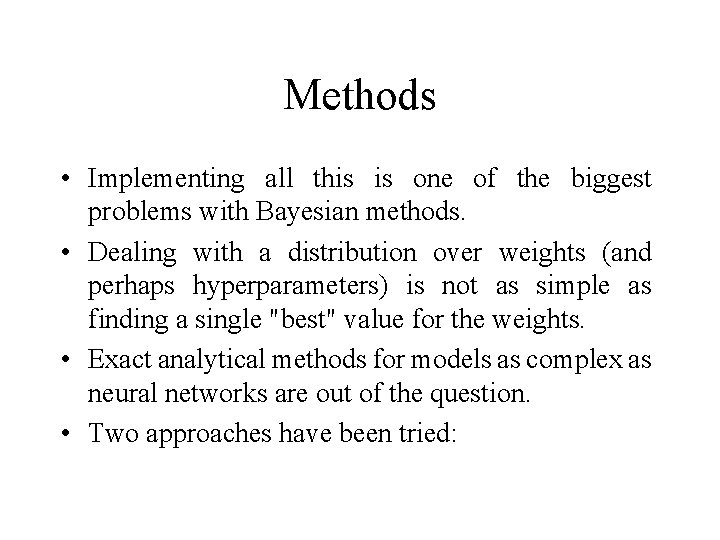Methods • Implementing all this is one of the biggest problems with Bayesian methods. • Dealing with a distribution over weights (and perhaps hyperparameters) is not as simple as finding a single "best" value for the weights. • Exact analytical methods for models as complex as neural networks are out of the question. • Two approaches have been tried:Methods · Find the weights/hyperparameters that are most probable, using methods similar to conventional training (with regularization), and then approximate the distribution over weights using information available at this maximum. • Use a Monte Carlo method to sample from the distribution over weights. The most efficient implementations of this use dynamical Monte Carlo methods whose operation resembles that of backprop with momentum.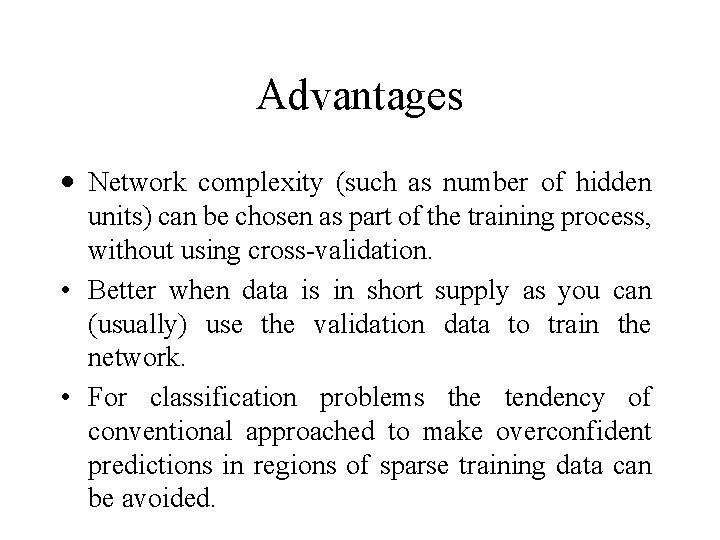Advantages · Network complexity (such as number of hidden units) can be chosen as part of the training process, without using cross-validation. • Better when data is in short supply as you can (usually) use the validation data to train the network. • For classification problems the tendency of conventional approached to make overconfident predictions in regions of sparse training data can be avoided.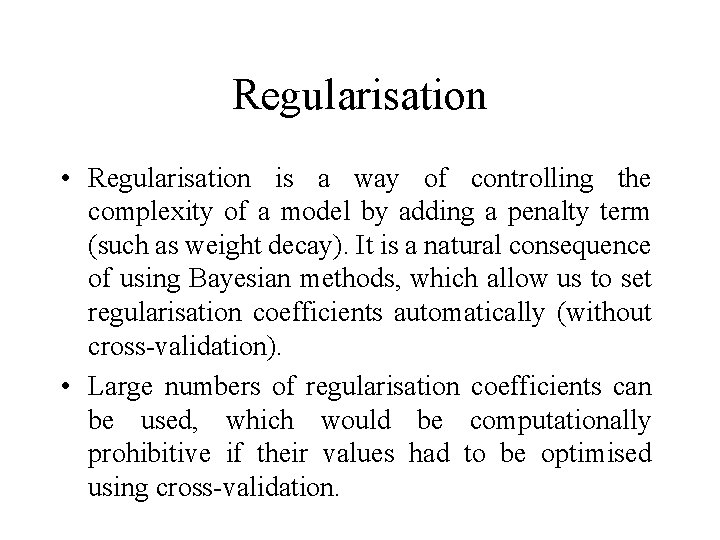Regularisation • Regularisation is a way of controlling the complexity of a model by adding a penalty term (such as weight decay). It is a natural consequence of using Bayesian methods, which allow us to set regularisation coefficients automatically (without cross-validation). • Large numbers of regularisation coefficients can be used, which would be computationally prohibitive if their values had to be optimised using cross-validation.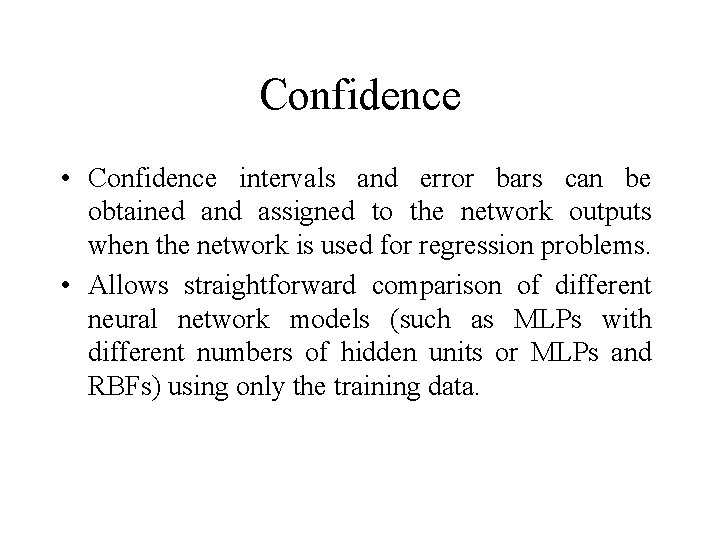Confidence • Confidence intervals and error bars can be obtained and assigned to the network outputs when the network is used for regression problems. • Allows straightforward comparison of different neural network models (such as MLPs with different numbers of hidden units or MLPs and RBFs) using only the training data.Advantages · Guidance is provided on where in the input space to seek new data (active learning allows us to determine where to sample the training data next). • Relative importance of inputs can be investigated (Automatic Relevance Detection) • Very successful in certain domains • Theoretically the most powerful method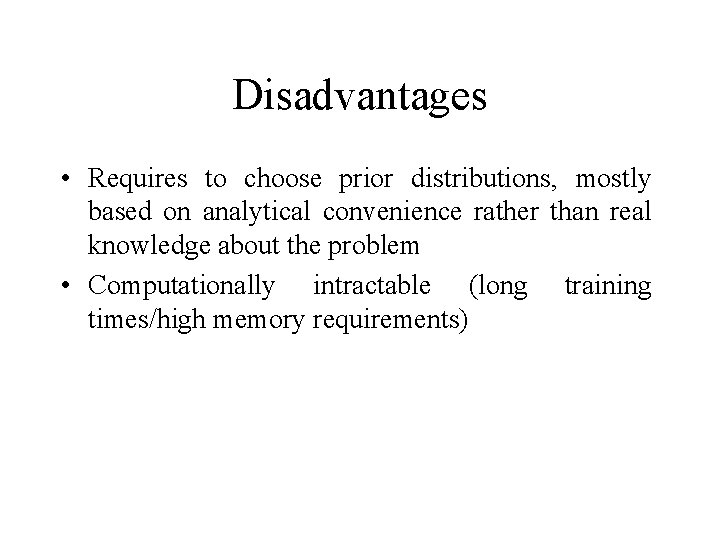Disadvantages • Requires to choose prior distributions, mostly based on analytical convenience rather than real knowledge about the problem • Computationally intractable (long training times/high memory requirements)Summary · In practice, Bayesian networks often outperform standard networks (such as MLPs trained with backpropagation). • However, there are several unresolved issues (such as how best to choose the priors) and more research is needed • Bayesian networks are computationally intensive and therefore take a long time to train.